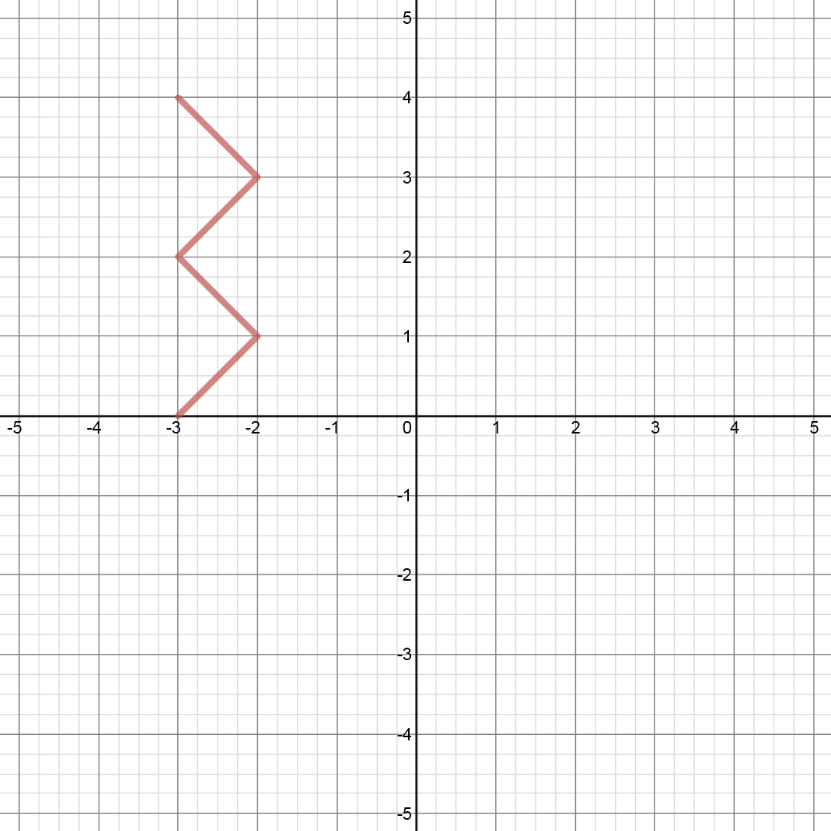# Inverse functions

### Inverse functions

An inverse function is a function that reverses all the operations of another function. Therefore, an inverse function has all the points of another function, except that the x and y values are reversed.

#### Lessons

• Introduction

• What is "inverse", and what does "inverse" do to a function?
• Inverse: switch "x" and "y"
• Inverse: reflect the original function in the line "y = x"

• 1.
Graph an inverse
Given the graph of $y = f\left( x \right)$ as shown,a)
Sketch the graph of the inverse $y = {f^{ - 1}}\left( x \right)$ in 2 ways:
i) by reflecting $f\left( x \right)$ in the line $y = x$
ii) by switching the x and y coordinates for each point on $f\left( x \right)$

b)
Is $f\left( x \right)$ a function?
Is ${f^{ - 1}}\left( x \right)$ a function?

• 2.
Inverse of a Quadratic Function
Consider the quadratic function: $f(x) = (x+4)^2 + 2$
a)
Graph the function $f\left( x \right)$ and state the domain and range.

b)
Graph the inverse ${f^{ - 1}}\left( x \right)$ and state the domain and range.

c)
Is ${f^{ - 1}}\left( x \right)$ a function?
If not, describe how to restrict the domain of $f\left( x \right)$ so that the inverse of $f\left( x \right)$ can be a function.

• 3.
Determine the equation of the inverse.
Algebraically determine the equation of the inverse ${f^{ - 1}}\left( x \right)$, given:
a)
$f\left( x \right) = - 5x + 4$

b)
$f\left( x \right) = {\left( {7x - 8} \right)^3} - 1$

c)
$f\left( x \right) = \frac{{3x}}{{2 + x}}$